# SOF - IMO PDF Sample Papers for Class 7

Resources:

Class 7 sample paper & practice questions for International Mathematics Olympiad (IMO) level 1 are given below. Syllabus for level 1 is also mentioned for these exams. You can refer these sample paper & quiz for preparing for the exam.Q.1 Q.2 Q.3 Q.4 Q.5 Q.6 Q.7 Q.8 Q.9 Q.10
 Q.1 For a fixed base, if the exponent decreases by 1 then what will be the number? a) One-tenth of the previous number. b) Ten times of the previous number. c) Hundredth of the previous number. d) Hundred times of the previous number.
 Q.2 A lends Rs. 3500 to B at 10% p.a. and B lends the same sum to C at 11.5% p.a. Find the gain of B (in Rs.) in a period of 3 years. a) Rs. 154.50 b) Rs. 155.50 c) Rs. 157.50 d) Rs. 156.50
 Q.3 A triangle can be constructed by taking two of its angles as: a) 90 degree, 90 degrees b) 135 degree, 45 degrees c) 70 degree, 115 degrees d) 110 degree, 40 degrees
 Q.4 What is the lowest form of 12/30? a) 1/5 b) 1/2 c) 2/5 d) 6/15
 Q.5 Which of the following image are reflections of each other? a)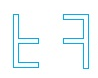b)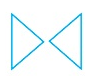c)d)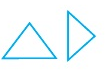Q.6 In the following question, find out which of the answer figures (1), (2), (3) and (4) completes the figure matrix?Select a suitable figure from the four alternatives that would complete the figure matrix.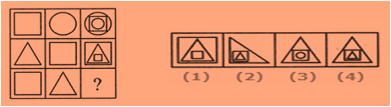a) 1 b) 2 c) 3 d) 4
 Q.7 Name the solid that has two opposite identical faces and other faces as parallelogram. a) Pyramid b) Prism c) Sphere d) Cone
 Q.8 Factorize. -4h2 + 81. a) (9 + 3h)(9 - 3h) b) (9 + 2h)(9 - 2h) c) 16h - 45 d) None of above
 Q.9 The bar graph given below shows the sales of books (in thousand number) from six branches of a publishing company during two consecutive years 2000 and 2001. Total sales of branch B6 for both the years is what percent of the total sales of branches B3 for both the years?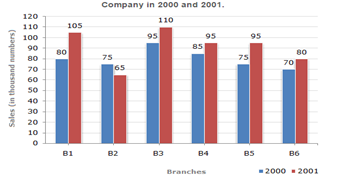a) 73.17% b) 75.55% c) 71.11% d) 68.54%
 Q.10 Choose from the alternatives (a), (b), (c) and (d), the boxes that are similar to the box that will be formed.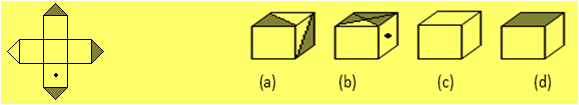a) a b) b c) c d) dSample PDF of SOF - International Mathematics Olympiad (IMO) PDF Sample Papers for Class 7: